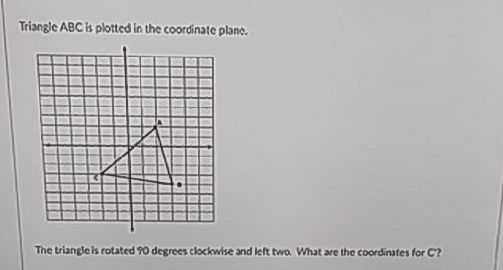### Still have math questions?Triangle $$A B C$$ is plotted in the coordinate plane. The triande is rotated $$\ 0$$ degrees clockwise and left two. What are the coordirafes for CF?